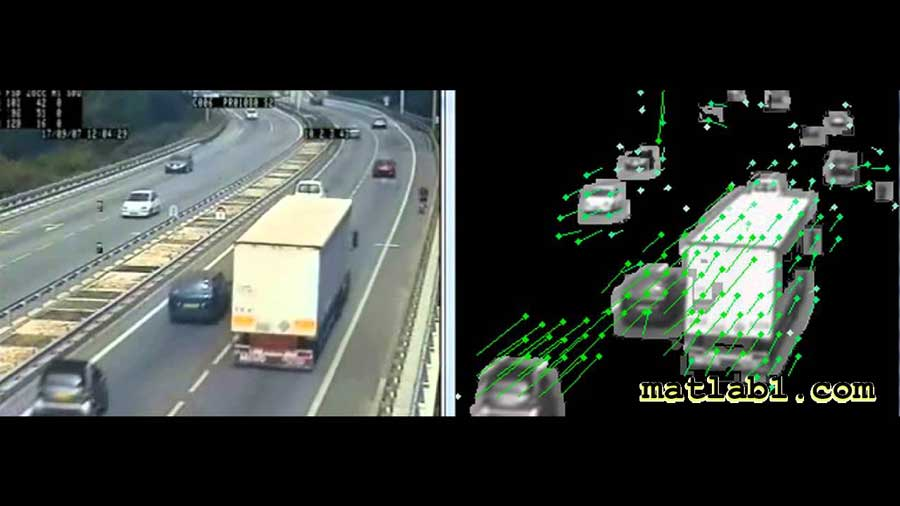# Optical Flow in MATLAB for computer vision

## Description

In this Online Courses, you will learn how to implement optical flow in MATLAB.Optical Flow MATLAB computer vision

In recent years, the optical flow has found many applications in machine vision.
In general, obvious motion changes in the brightness of an image are said to be due to the movement of targets. However, changes in the intensity of motion are similar to changes in ambient light or shadow but do not include optical flow.

The shorter the time between taking two consecutive images, the more similar the images are to each other, in which case the changes can be displayed in vector format proportional to the speed of the image coordinate device. In this case, the movement of the camera between the images is equal to the speed and the changes in the image mapping the speed of the environment. This small motion in the image is called optical flow.Optical flow is usually considered in two ways, in the dense state, where the flow vector is calculated for each pixel, and in the feature-based state, which is calculated for the specific purposes for which it is intended.
If we want to calculate optical flow in a spherical model, we must either the first sample the environment as spherical space and then calculate optical flow (which is difficult and requires a lot of calculations), or optical flow is calculated in ordinary space and the result is through The Jacobin matrix is transferred to the spherical space.

## Content title:

Definition of optical flow

How many frames are needed to calculate Optical flow?

Example of a Hamburg taxi and the corresponding optical flow image

Example 2 Optical flow image

Example 3 Optical flow image

Example 4 (garden dataset) and the optical flow image

In which areas of the image, the optical flow faces a problem?

Display sample video and optical flow vectors on it

Examine situations in the video where the optical flow gives a bad result?

The distance and proximity of the object to the camera and its effect on optical flow

The difference between the light intensity of the object and the background and its effect on the calculation of optical flow

Optical flow applications

Horn & Schunck method

What is the main idea in optical flow?

Mathematical relations of the main idea of ​​optical flow

The difference between motion and optical flow

Is every motion an optical flow?

Impact of lightening on the calculation of optical flow

When does Lucas-Kanade make a mistake?

When does Keypoint Mataching enter the optical flow calculation?

When does Region-Based matching enter the optical flow calculation?

When does the Gradient Constant enter the optical flow calculation?

Start MATLAB optical flow programming

OpticalFlow command

the MATLAB program draws a random optical flow

The concept of optical flow numbers

Draw optical flow vectors

DecimationFactor option

ScaleFactor option

OpticalFlowHS command

The second MATLAB program

Explain the output parameters of the video reading command

Calculation of the frame width

Calculation of the frame height

Calculation of the total video frames

HasFrame command

EstimateFlow command

Optical flow output fields

Concepts Vx, Vy, Orientation and Magnitude

Calculate the minimum and maximum optical flow size

View optical flow on the video frame

Place a condition on the size of the optical flow

Morphological operation on the image

Object tracking based on optical flow

Place the bounding box on moving objects

InsertShape command

Smoothness parameter

MaxIteration parameter

VelocityDifference parameter

What are the conditions for stopping optical flow?

How to control the stop conditions?

Impact of each parameter in an example MATLAB application

The opticalFlowLK command

NoiseThreshold option

The Lucas-kanade method is a derivative of the Gaussian method

The opticalFlowLKDoG command

NumFrames option

ImageFilterSigma option